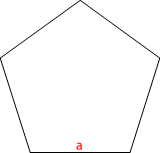# Pentagon Area Calculator

In Euclidean geometry, a regular polygon is a polygon that is equiangular (all angles are equal in measure) and equilateral (all sides have the same length). Regular polygons may be convex or star (a type of non-convex polygon). As the number of sides of a regular polygon increases, the polygon increasingly approximates a circle if the perimeter is fixed, or a regular apeirogon, if the edge length is fixed.Side (a):